Study Guide

# Definite Integrals - Properties of Definite Integrals

## Properties of Definite Integrals

There are a lot of useful rules for how to combine integrals, combine integrands, and play with the limits of integration. For some functions there are shortcuts to integration.

For this whole section, assume that f(x) is an integrable function.

### Warming Up

Some properties we can see by looking at graphs.

• The integral of the zero function is 0. In symbols, no matter what a and b are,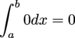It doesn't matter what the interval is, because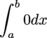is the area of a rectangle with height 0. However long the rectangle is, a rectangle with height 0 also has area 0.

• If we integrate an integrable function on a length 0 interval, we get 0. In symbols,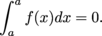The expression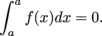describes the area between f and the x-axis on an interval with length 0. An area with width 0 must be 0.

• If c is a constant, then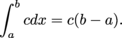The area between the constant function c and the x-axis is a rectangle with width ba  and "height" c. The weighted area of this rectangle is c(b a).

• We can change the variable of integration at will: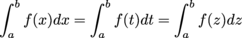When we change the variable of integration, all we're changing is the labeling on the horizontal axis. The shape of the function (and the weighted area between the function and the horizontal axis) won't change.

• ### Single-Function Properties

These properties require a little more explanation. We're still assuming f is an integrable function.

• Let c be a constant. Then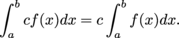As an example, let f = x on [0, b] and let c = 3.

When we stretch f by 3 we don't change the base of the triangle, but we do stretch the height by 3. We also multiply the area by 3.

When we rewrite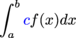as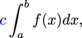we say we're "pulling out the constant" or "pulling the constant out of the integral."

• We can switch the limits of integration if we also switch the sign: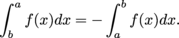We've been focusing on integrals from a to b where a < b. If f is non-negative then as we accumulate area from left to right, we weight the area positively. As we accumulate area from right to left, we weight it negatively.

•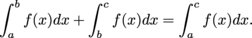The integral of f on [a, b] is the weighted area between f and the x-axis on [a, b]. The integral of f on [b,c] is the weighted area between f and the x-axis on [b, c]. When we add these weighted areas, we get the weighted area between f and the x-axis on [a, c], which is the integral of f on [a, c]. When a,b,c don't fall nicely in order, this is still true.

• If f (x) is an even function then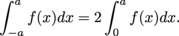If f is even then it's reflected across the y-axis.This means whatever area is on one side of the graph is also on the other side of the graph. The weighted area between f and the x-axis on [-a, a] is then double the weighted area between f and the x-axis on [0, a].

• If f is an odd function then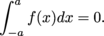You can see that the area between f and the horizontal axis is the same on both sides, but on one side the area is above the x-axis and on the other it's below the x-axis. Odd functions will always work this way. If we integrate from -a to a the weighted areas above and below the x-axis will cancel each other out.

• ### Talking About Two Functions

Now another function is going to join the party. Assume both f and g are integrable functions.

• The integral of a sum is the sum of the integrals. In symbols,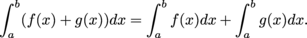Also, the integral of a difference is the difference of the integrals: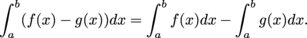We've taken whatever weighted area was between g and the x-axis, and stuck that on top of f. If g is negative, in some places we may actually be subtracting area from f. The integral of (f + g) is the integral of f plus the integral of g.

• If f (x) is smaller than g(x), the integral of f is smaller than the integral of g. In symbols, if

f (x) < g(x) for all x in [a, b]

then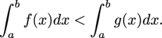• If both f and g are positive, it's clear that there's more area between g and the x-axis than between f and the x-axis:
• If both f and g are negative, the weighted area between g and the x-axis is closer to 0 than the weighted area between f and the x-axis, so we still have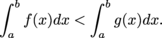• If f is negative and g is positive, then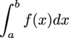is negative and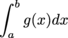is positive.
• If f and g are sometimes negative and sometimes positive, we can split the interval [a, b] into sub-intervals on which one of the previous conditions holds, and go from there.
• Let m and M be constants. If mf(x) ≤ M on [a, b], then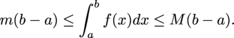This is using the last property. We're comparing f to the constant functions m and M. If mf (x)on [a, b], then we know from the previous property thatSince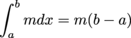, we have half the inequality explained.

Similarly, if f (x) ≤ M on [a,b] then we know from the previous property that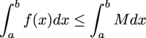and we know that .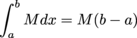### Sample Problem

If f (x) < 2x, then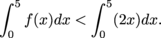We can find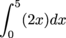by looking at the graph and getting the area of the triangle: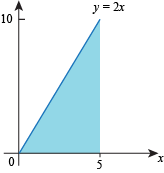We conclude that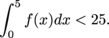### Sample Problem

If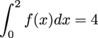and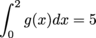, then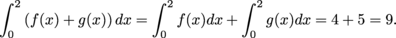Be Careful: The integral of a product is not necessarily the product of the integrals.

• ### Thinking Backwards

"Thinking backwards" shows up in a lot of places. That means it must be important! Here, "thinking backwards" means instead of using a known integral value to evaluate an expression, we'll work backwards from an equation to find the integral value.

Think of the integral you're looking for as a unit. Instead of solving for x, you'll be solving for something like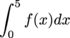. If your equation contains a slightly different integral than the one you want, use the properties to manipulate the integral until it's what you're looking for.